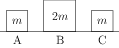# Elastic and Inelastic Collisions

## Problems from IIT JEE

Problem (IIT JEE 2009):Three objects A, B and C are kept in a straight line on a frictionless horizontal surface. These have masses $m$, $2m$ and $m$, respectively. The object A moves towards B with a speed 9 m/s and makes an elastic collision with it. Thereafter, B makes completely inelastic collision with C. All motions occur on the same straight line. Find the final speed (in m/s) of the object C.

Solution: Let $v_A$ and $v_B$ be the velocities of A and B after an elastic collision. The conservation of linear momentum gives, \begin{align} \label{rfa:eqn:1} m\times 9+0=mv_A+2mv_B. \end{align} The conservation of energy gives, \begin{align} \label{rfa:eqn:2} \tfrac{1}{2}m\times 9^2+0=\tfrac{1}{2}mv_A^2+\tfrac{1}{2}\,(2m)v_B^2. \end{align} Solve above equations to get $v_B={6}\;\mathrm{m/s}$. The collision between B and C is completely inelastic i.e., after the collision both particles coalesce and move with a common speed, say $v_C$. The conservation of linear momentum gives, \begin{align} \label{rfa:eqn:3} (2m)\times 6+0=(2m+m)v_C. \end{align} Solve to get $v_C=4\;\mathrm{m/s}$.

Problem (IIT JEE 2007):

Statement 1: In an elastic collision between two bodies, the relative speed of the bodies after collision is equal to the relative speed before the collision.

Statement 2: In an elastic collision, the linear momentum of the system is conserved.

1. Statement 1 is true, statement 2 is true; statement 2 is a correct explanation for statement 1.
2. Statement 1 is true, statement 2 is true; statement 2 is not a correct explanation for statement 1.
3. Statement 1 is true, statement 2 is false.
4. Statement 1 is false, statement 2 is true.

Solution: Let $v_s$ be the velocity of separation and $v_a$ be the velocity of approach. The $v_s$ and $v_a$ are related by, $v_s=e v_a$, where $e$ is the coefficient of restitution. The energy conservation in elastic collision gives $e=1$.